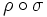# Class-preserving implies linearly pushforwardable

This article gives the statement and possibly, proof, of an implication relation between two automorphism properties. That is, it states that every automorphism satisfying the first automorphism property (i.e., class-preserving automorphism) must also satisfy the second automorphism property (i.e., linearly pushforwardable automorphism)
View all automorphism property implications | View all automorphism property non-implications
Get more facts about class-preserving automorphism|Get more facts about linearly pushforwardable automorphism

## Statement

Suppose$G$ is a group and$k$ is a Class-determining field (?) for$G$. Then, any Class-preserving automorphism (?) of$G$ is linearly pushforwardable.

## Definitions used

### Class-determining field

Further information: class-determining field

A field$k$ is termed class-determining for a group$G$ if, given any two finite-dimensional linear representations of$G$ on a vector space$V$ over$k$, say$\rho_1, \rho_2: G \to GL(V)$, such that for every$g \in G$, the elements$\rho_1(g)$ and$\rho_2(g)$ are conjugate inside$GL(V)$, we can conclude that$\rho_1$ and$\rho_2$ are equivalent.

In other words, the conjugacy classes in$GL(V)$ of the images of elements in$G$, determine the representation.

Note that what this statement really says is that if two representations are conjugate at every element of$G$, they are equivalent, or globally conjugate.

For a finite group, any field whose characteristic does not divide the order of the group is a character-determining field, and hence a class-determining field.

### Class-preserving automorphism

Further information: Class-preserving automorphism

An automorphism of a group is termed class-preserving if it sends each element to within its conjugacy class.

### Linearly pushforwardable automorphism

Further information: Linearly pushforwardable automorphism

An automorphism$\sigma$ of a group$G$ is termed linearly pushforwardable over a field$k$ if for any finite-dimensional linear representation$\rho:G \to GL(V)$, there exists an element$a \in GL(V)$ such that if$c_a$ denotes conjugation by$a$, then$\rho \circ \sigma = c_a \circ \rho$. In other words, for any$g \in G$:$\rho(\sigma(g)) = a\sigma(g)a^{-1}$

## Proof

Given: A group$G$, a class-determining field$k$ for$G$, a class-preserving automorphism$\sigma$ of$G$, and a finite-dimensional linear representation$\rho: G \to GL(V)$

To prove: There exists$a \in GL(V)$ such that$\rho(\sigma(g)) = a \sigma(g)a^{-1}$ for every$g$.

Proof: Observe first that$\sigma$ and$\rho \circ \sigma$ are both linear representations of$G$, since$\sigma$ is an automorphism. Further, since$\sigma$ is class-preserving, it is true that for any$g \in G$, there exists$h \in G$ such that$\sigma(g) = hgh^{-1}$. We thus obtain:$\rho(\sigma(g)) = \rho(h)\rho(g)\rho(h)^{-1}$

In other words,$\rho(\sigma(g))$ and$\rho(g)$ are conjugate in$GL(V)$.

Now, by the definition of class-determining field, we see that$\rho \circ \sigma$ and$\rho$ are equivalent linear representations. Thus, there exists$a \in GL(V)$ such that for every$g \in G$:$\rho(\sigma(g)) = a \sigma(g)a^{-1}$